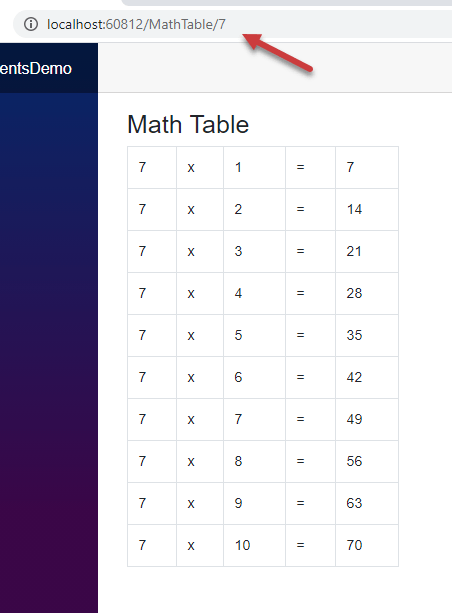# Blazor 组件入门指南

Blazor 应用程序是组件的组合，这些组件不仅负责呈现用户界面，还协同工作以促进用户交互。它们是 Blazor 应用程序的主要构建块，大多数 Blazor 功能都是围绕组件展开的。在本教程中，我将向您详细介绍组件，并向您展示在 Blazor 应用程序中创建和使用组件的多种技术。.

## Blazor 组件概述

Blazor 组件是 UI 的一个自包含部分，例如一个页面、一个侧边栏菜单、一个联系人表单或仪表盘小工具等。它包括用于呈现 UI 的 HTML 标签和用于处理数据或处理用户事件的 C# 代码。组件可以相互嵌套，也可以在项目中重用，甚至可以跨多个项目重用。Blazor 组件是作为 Razor 组件实现的，这正是它们使用 Razor 语法并具有 .razor 文件扩展名的原因。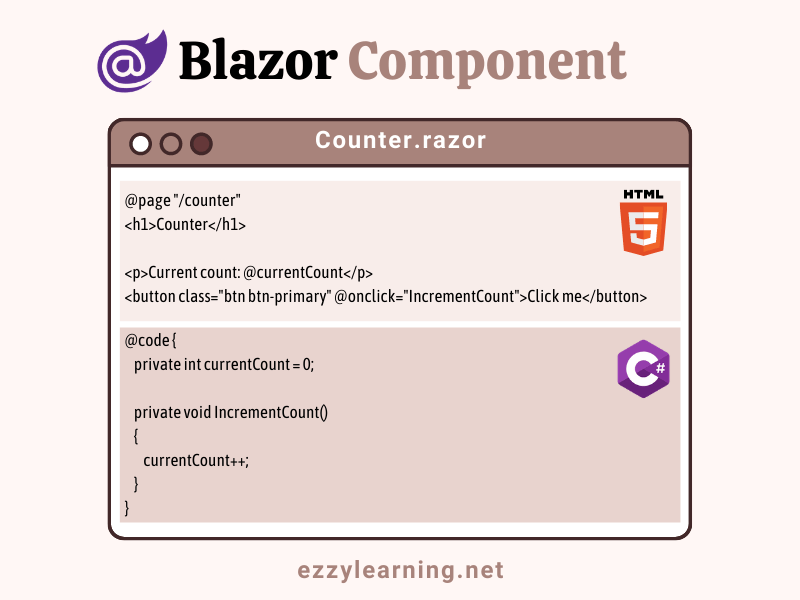``````@page "/counter"

<h1>Counter</h1>

<p>Current count: @currentCount</p>

<button class="btn btn-primary" @onclick="IncrementCount">Click me</button>

@code {
private int currentCount = 0;

private void IncrementCount()
{
currentCount++;
}
}
``````

``````@page "/counter"
``````

``````@page "/counter"
@page "/mycounter"
``````

``````<p>Current count: @currentCount</p>
``````

``````<button class="btn btn-primary" @onclick="IncrementCount">Click me</button>
``````

``````@code {
private int currentCount = 0;

private void IncrementCount()
{
currentCount++;
}
}
``````

## 在 Visual Studio 2019 中创建 Blazor 组件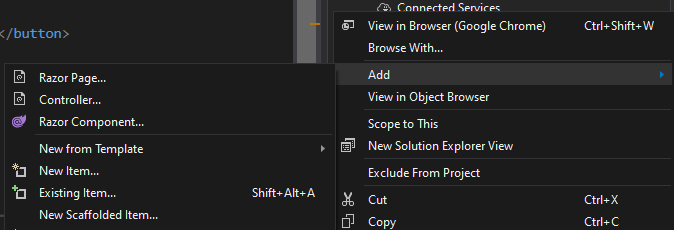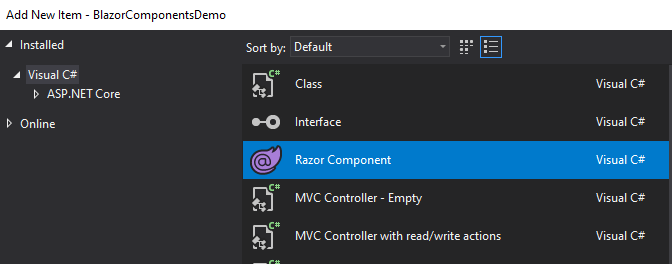Calculator.razor

``````@page "/calculator"

<h3>Calculator</h3>

<div class="form-group">
<label for="number1">Number 1</label>
<input type="number" class="form-control" id="number1" @bind="number1">
</div>
<div class="form-group">
<label for="number2">Number 2</label>
<input type="number" class="form-control" id="number2"  @bind="number2">
</div>
<div class="form-group">
<label><b>Total: </b>@total</label>
</div>

<button class="btn btn-primary" @onclick="Calculate">Calculate</button>

@code {
private int number1 = 0;
private int number2 = 0;
private int total = 0;

private void Calculate()
{
total = number1 + number2;
}
}
``````

HTML 标记中有两个输入框，它们使用 @bind 属性来绑定 number1 和 number2 变量：

``````<input type="number" class="form-control" id="number1" @bind="number1">
``````

``````<label><b>Total: </b>@total</label>
``````

``````<button class="btn btn-primary" @onclick="Calculate">Calculate</button>
``````

``````<li class="nav-item px-3">
<span class="oi oi-calculator" aria-hidden="true"></span> Calculator
</li>
``````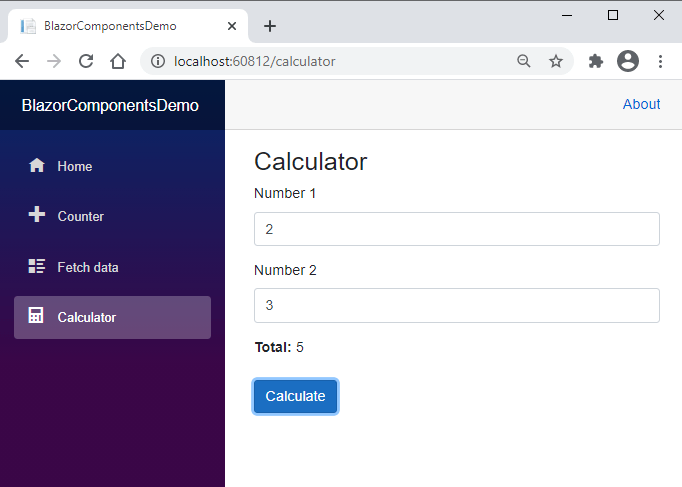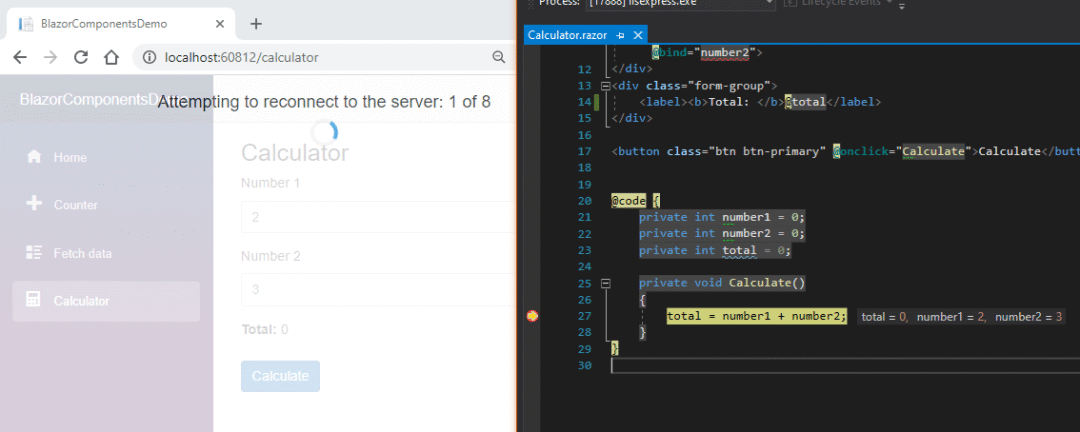## 拆分 Blazor 组件中的标签和代码

### §使用基类拆分组件

CalculatorBase.cs

``````public class CalculatorBase : ComponentBase
{
private int number1 = 0;
private int number2 = 0;
private int total = 0;

private void Calculate()
{
total = number1 + number2;
}
}
``````

Calculator.razor

``````@page "/calculator"
@inherits CalculatorBase

<h3>Calculator</h3>

<div class="form-group">
<label for="number1">Number 1</label>
<input type="number" class="form-control" id="number1" @bind="number1">
</div>
<div class="form-group">
<label for="number2">Number 2</label>
<input type="number" class="form-control" id="number2"  @bind="number2">
</div>
<div class="form-group">
<label><b>Total: </b>@total</label>
</div>

<button class="btn btn-primary" @onclick="Calculate">Calculate</button>

@code {

}
````````````public%20class%20CalculatorBase%20:%20ComponentBase
{
%20%20%20%20public%20int%20number1%20=%200;
%20%20%20%20public%20int%20number2%20=%200;
%20%20%20%20public%20int%20total%20=%200;
%20
%20%20%20%20public%20void%20Calculate()
%20%20%20%20{
%20%20%20%20%20%20%20%20total%20=%20number1%20+%20number2;
%20%20%20%20}
}
``````

### §使用部分类拆分组件

Blazor%20组件生成为部分类，这意味着我们可以创建一个与我们的组件同名的 partial 类，并将所有%20C#%20代码移动到该部分类中。然后，此部分类将成为代码隐藏文件，该文件中声明的字段和属性可在%20Blazor%20组件中直接使用。让我们创建一个类 Calculator.razor.cs，并将%20Calculator%20的代码放在这个新类中。

Calculator.razor.cs

``````public%20partial%20class%20Calculator
{
%20%20%20%20public%20int%20number1%20=%200;
%20%20%20%20public%20int%20number2%20=%200;
%20%20%20%20public%20int%20total%20=%200;
%20
%20%20%20%20public%20void%20Calculate()
%20%20%20%20{
%20%20%20%20%20%20%20%20total%20=%20number1%20+%20number2;
%20%20%20%20}
}
``````

## 创建和使用子组件

Blazor%20子组件是没有%20@page%20指令的组件。这些组件可以使用标准的%20HTML%20语法包含在其他组件中。然后，我们可以通过在页面上添加组件来构建复杂的%20UI，我们甚至可以在同一个页面上添加同一子组件的多个实例。如果一个子组件可能在多个父组件或页面中重复使用，那么最好将它放在 Shared 文件夹中。让我们在 Shared 文件夹中创建一个简单的 Heading.razor 子组件，并在其中添加以下代码。

``````<h3>Calculator</h3>
``````

Calculator.razor

``````@page%20"/calculator"
@inherits%20CalculatorBase
%20
%20
<div%20class="form-group">
%20%20%20%20<label%20for="number1">Number%201</label>
%20%20%20%20<input%20type="number"%20class="form-control"%20id="number1"%20@bind="number1">
</div>
<div%20class="form-group">
%20%20%20%20<label%20for="number2">Number%202</label>
%20%20%20%20<input%20type="number"%20class="form-control"%20id="number2"%20%20@bind="number2">
</div>
<div%20class="form-group">
%20%20%20%20<label><b>Total:%20</b>@total</label>%20
</div>
%20
<button%20class="btn%20btn-primary"%20@onclick="Calculate">Calculate</button>
``````

``````<Heading%20/>
``````

## 自定义带参数的 Blazor 组件

``````<h3>@Title</h3>

@code {
[Parameter]
public string Title { get; set; } = "Default Title";
}
``````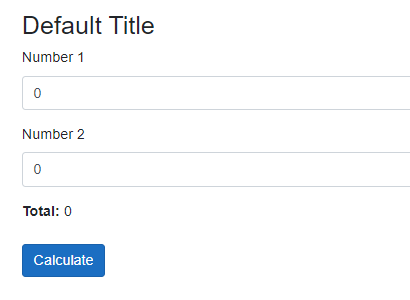Visual Studio 智能感知也可以提示组件参数，因此我们不需要记忆这些参数，这很有用。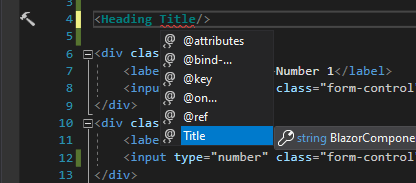``````<Heading Title="Calculator" />
``````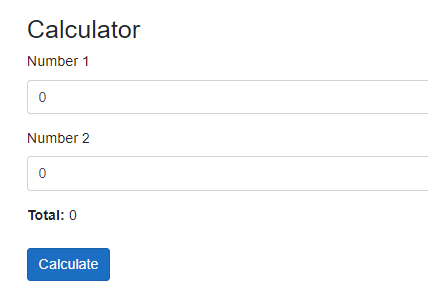CalculatorTotal.razor

``````<label><b>Total: </b>@Total</label>

@code {
[Parameter]
public int Total { get; set; }
}
``````

Calculator.razor

``````@page "/calculator"

<div class="form-group">
<label for="number1">Number 1</label>
<input type="number" class="form-control" id="number1" @bind="number1">
</div>
<div class="form-group">
<label for="number2">Number 2</label>
<input type="number" class="form-control" id="number2" @bind="number2">
</div>
<div class="form-group">
<CalculatorTotal Total="@total"/>
</div>

<button class="btn btn-primary" @onclick="Calculate">Calculate</button>
``````

## 将路由参数传递给 Blazor 组件

Blazor 组件还可以接受在 @page 指令中提供的来自路由模板的参数。路由器使用路由参数自动填充相应的组件参数。

MathTable.razor

``````@page "/MathTable/{number:int}"

<h3>Math Table</h3>

<table class="table table-bordered w-25">
@for (int i = 1; i <= 10; i++)
{
<tr>
<td>@Number</td>
<td>x</td>
<td>@i</td>
<td>=</td>
<td>@(Number * i)</td>
</tr>
}
</table>

@code {
[Parameter]
public int Number { get; set; }
}
``````

``````@page "/MathTable/{number:int}"
``````

``````[Parameter]
public int Number { get; set; }
``````